#Reasoning Questions: Coding And Decoding(New Pattern) Set 30

Hello Aspirants.

Welcome to Online Reasoning Section in AffairsCloud.com. Here we are creating question sample in Coding and Decoding, which is common for all competitive exams. We have included Some questions that are repeatedly asked in bank exams!!!

(Direction 1 – 5): In each of the following questions two rows of numbers are given. The resultant number in each row is to be worked out separately based on the following rules and the question below the rows of numbers are to be answered. The operations of numbers progress from left to right.
Rules:
i) If the odd number is following by a two digit even number then they are added to each other.
ii) If the odd number is following by a two digit odd number then the second number is to be subtracted from the first number.
iii) If the even number is following by a number which is a perfect square of a number then the second number is to be divided by the first number.
iv) If the even number is following by a two digit even number then the first number is to be multiplied by the second number.

1.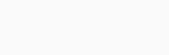What will be the answer if the resultant of the second row is subtracted from the resultant of the first row?
1) 144
2) 92
3) 129
4) 112
5) None of these
Solution:i) In first row 55 is an odd number and 45 is a two digit odd no. so apply condition 2
→ 55 – 45 = 10
Now 10 is an even no. and next 12 is a two digit even no. so apply condition 4
→ 10 × 12 = 120 and 120 is the resultant of 1st row.
ii) In 2nd row 25 is an odd no. and 10 is a two digit even no. so condition 1 is applied
→ 25 + 10 = 35
Now 25 is an odd no. and next 33 is a two digit odd no. so apply condition 2
→ 35 – 33 = 2
Now 2 is an even number and next 16 is a perfect square so apply condition 3
→ 16 ÷ 2 = 8 and 8 is the resultant of the second row
Now subtract resultant of 2nd from resultant of 1st row = 120 – 8 = 112.

2.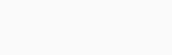What is the answer if the resultant of the first row is multiplied by the resultant of the second row?
1) 19
2) 30
3) 16
4) 20
5) None of these
Solution:
i) In first row 39 is an odd number and 35 is a two digit odd no. so apply condition 2
→ 39 – 35 = 4
Now 4 is an even no. and next 10 is a two digit even number so apply condition 4
→ 4 × 10 = 40
Now 40 is an even number and next 400 is a perfect square so apply condition 3
→ 400 ÷ 40 = 10 and 10 is the resultant of 1st row.
ii) In 2nd row 4 is an even no. and 12 is a two digit even no. so condition 4 is applied
→ 4 × 12 = 48
Now 48 is an even no. and next 144 is a perfect square so apply condition 3
→ 144 ÷ 48 = 3 and 3 is the resultant of the second row
Now multiply resultant of 1st and resultant of 2nd row = 10 × 3= 30.

3.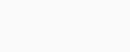What is the sum of the resultant of first row and second row?
1) 22
2) 20
3) 18
4) 42
5) None of these
Solution:
i) In first row 30 is an even number and 60 is a two digit even no. so apply condition 4
→ 30 × 60 = 1800
Now 1800 is an even no. and next 3600 is a perfect square so apply condition 3
→ 3600 ÷ 1800 = 2 and 2 is the resultant of 1st row.
ii) In 2nd row 15 is an odd no. and 22 is a two digit even no. so condition 1 is applied
→ 15 + 22 = 37
Now 37 is an odd no. and next 19 is a two digit odd no. so apply condition 2
→ 37 – 19 = 18 and 18 is the resultant of the second row
Now sum of resultant of 1st and 2nd row = 2 + 18 = 20.

4.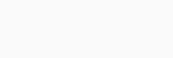What is the difference between the resultant of the first row of numbers and the second row of numbers?
1) 20
2) 210
3) 69
4) 54
5) None of these
Solution:
i) In first row 6 is an even number and 36 is a perfect square so apply condition 3
→ 36 ÷ 6 = 6
Now 6 is an even no. and next 36 is a perfect square so apply condition 3
→ 36 ÷ 6 = 6
Now 6 is an even no. and next 12 is a two digit even number so apply condition 4
→ 6 × 12 = 72 and 72 is the resultant of 1st row.
ii) In 2nd row 15 is an odd no. and 11 is a two digit odd no. so condition 2 is applied
→ 17 – 13 = 4
Now 4 is an even number and next 12 is a two digit even no. so apply condition 4
→ 4 × 12 = 48
Now 48 is an even number and 144 is a perfect square so apply condition 3
→ 144 ÷ 48 = 3 and 3 is the resultant of the second row
Now difference of resultant of 1st and 2nd row = 72 – 3 = 69.

5.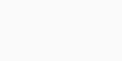What is the answer if the resultant of the first row is multiplied by the resultant row?
1) 248
2) 364
3) 192
4) 148
5) None of these
Solution:
i) In first row 21 is an odd no. and 19 is a two digit odd no. so apply condition 2
→ 21 – 19 = 2
Now 2 is an even no. and next 16 is a perfect square so apply condition 3
→ 16 ÷ 2 = 8 and 8 is the resultant of 1st row.
ii) In 2nd row 25 is an odd no. and 23 is a two digit odd no. so condition 2 is applied
→ 25 – 23 = 2
Now 2 is an even no. and next 12 is a two digit even no. so apply condition 4
→ 2 × 12 = 24 and 24 is the resultant of the second row.
Now product of resultant of 1st and 2nd row = 8 × 24 = 192.

(Directions 6 – 10): Given below are the codes for the digits/symbols. Study the conditions given below and answer the questions that follow.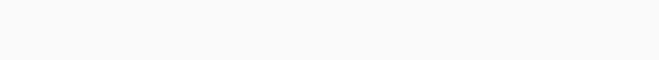Conditions:
(1) If both the left most and the right most elements are symbols, then the codes for the first two elements get interchanged between them and the codes for the last two elements get interchanged between them.
(2) If the left most element is a symbol and the right most element is an even digit then the code for both will be Y.
(3) If the left most element is an odd digit and the right most element is a symbol then the codes of these two elements got interchanged.
(4) If the left most element is an odd digit and the right most element is an even digit then the code for both will be W.
(5) If the left most element in the group is an even digit and the right most element is an odd digit then reverse the order of the code for the group.

1. 836@497
1) PDECHFA
2) BFHCEDA
4) XDECHFX
5) YDECHFY
Solution:
It satisfies condition (5).
If the left most element in the group is an even digit and the right most element is an odd digit then reverse the order of the code for the group.
Hence, code for 836@497 is BFHCEDA.

2. 3#%7964
1) HEFBJGD
2) WGJBFEW
3) XGJBFEX
4) HGJBFED
5) None of these
Solution:
It satisfies condition (4).
If the left most element is an odd digit and the right most element is an even digit then the code for both will be W.
Hence, code for 3#%7964 is WGJBFEW.

3. \$4783&6
1) EKDABHQ
Solution:
It satisfies condition (2).
If the left most element is a symbol and the right most element is an even digit then the code for both will be Y.
Hence, code for \$4783&6 is YHBADKY.

4. 51&269@
1) YRKLEFY
2) ZRKLEFZ
3) XRKLEFX
4) CRKLEFT
5) None of these
Solution:
It satisfies condition (3).
If the left most element is an odd digit and the right most element is a symbol then the codes of these two elements got interchanged.
Hence, code for 51&269@ is CRKLEFT.

5. %8@521*
1) AJCTLNR
2) NACTLRJ
3) ZACTLRZ
4) YACTLRY
5) None of these